# Annualize Returns

The returns of investments are often expressed in different frequencies. For example, some financial advertisements might report the total return over the duration of the investment. Others financial products might report the average return over the past few years. However, when we want to compare the return of different investments, we need to make sure that they are expressed in the same frequency so that they are comparable.

One thing we can do, is to annualize returns. On this page, we provide the necessary formulas that explain how to calculate the annualized rate of return. At the bottom of this page, we also implement all these formulas in Excel to provide a simple annualized return calculator.

## Annualized return formula

How to calculate the annualized return? There are two ways of annualizing returns. First, we can simple divide or multiply the return to obtain the annualized return. Suppose, for example, that we have a 3-month return of 4%. In that case, we can just calculate the annual return as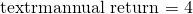In that case, the annualized return is 16%. This is referred to as the simple interest. It is important to note that, if you want to annualize volatility, you have to use a different formula.

There is, however, one caveat when using this approach, and it is called compound interest. If the return we receive every 3 months also generates interest in turn, i.e. it is reinvested, the actual return will be higher. In that the case, we should use the following formula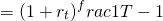where T, in years, is the frequency with which the return corresponds. In our case, T equals 3/12 or 0.25. Applying the above formula to our example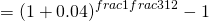in which case the annualized rate of return equals approximately 17%. The difference between both returns is 1%. This may sound like a small difference, but it compounds to a big difference after just a few years, as the following figure illustrates.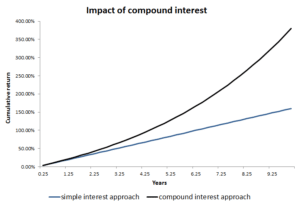Instead, consider an example where we have an average return on investment that equals 5% per half-year. At the same time, we know that it is a 3-year investment. What is the annualized return? Again, we can apply the same formulas

The simple interest annualized return equals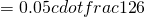which equals 12% per year. The annualized return based on compound interest, however, equals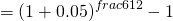or 12.36% annualized.

## Summary

We discussed how to annualize returns. This is an important concept as it allows investors toto compare the return to different invesments when they are expressed in different frequencies.